# 线圈半径对磁耦合谐振式无线电能传输特性的影响The Influence of the Coil Radius on Magnetic Coupled Resonant Wireless Power

• 全文下载: PDF(1548KB)    PP.118-126   DOI: 10.12677/JEE.2018.61013
• 下载量: 479  浏览量: 1,384

Transmission characteristics of Magnetic Coupled Resonant Wireless Power can be affected by the power frequency f, mutual inductance M between two coils and the influence of load RL, and the transmission power in strong coupling zone exists frequency division phenomenon. In this paper, we study the influence of the coil radius on the transmission properties of system, analysis of the two kinds of different radius of coil case system change with power frequency power transmission characteristics and efficiency characteristic of the changing with distance. The results are verified by experiments: in the case that the inductance of the resonant coil is constant, the resonant coil with a larger coil radius has a further effective transmission distance (critical coupling distance). For coil inherent in power frequency constant when the resonant frequency, with the increase of transmission distance, system transmission power were first increases then decreases, and the efficiency of the system decreases monotonously with the increase of distance, and at the same efficiency when the coil radius greater system can achieve further transmission distance.

1. 引言

2007年美国麻省理工学院(MIT)的Marin Soljacic教授课题组提出了磁耦合谐振式无线电能传输理论，并在传输距离为2 m时点亮60 W的灯泡  。磁耦合谐振式无线电能传输具有传输效率高、传输距离远、传输功率大以及对介质依赖性小等优点  ，为无线电能中等传输距离的研究指明了方向，因此磁耦合谐振式无线电能传输技术的研究立即受到世界各国科研学者的关注。

2. 系统模型和理论分析

2.1. 系统模型

2.2. 理论分析

2.2.1. 系统功率传输特性

$\left\{\begin{array}{l}{\stackrel{˙}{I}}_{1}=\frac{\left({R}_{D}+{R}_{L}+j{X}_{D}\right){\stackrel{˙}{V}}_{S}}{\left({R}_{2}+j{X}_{D}\right)\left({R}_{1}+j{X}_{S}\right)+{\left(\omega M\right)}^{2}}\\ {\stackrel{˙}{I}}_{2}=\frac{j\omega M{\stackrel{˙}{V}}_{S}}{\left({R}_{2}+j{X}_{D}\right)\left({R}_{1}+j{X}_{S}\right)+{\left(\omega M\right)}^{2}}\end{array}$ (1)

${X}_{D}={X}_{S}=X$ ,

${R}_{1}={R}_{S}+{R}_{0}$${R}_{2}={R}_{D}+{R}_{L}$${R}_{S}={R}_{D}=\frac{l}{4\text{π}a}\sqrt{\frac{{\mu }_{0}\omega }{2\sigma }}$

M为发射和接收线圈之间的互感。

${P}_{Load}={|{\stackrel{˙}{I}}_{2}|}^{2}{R}_{L}={|\frac{j\omega M{\stackrel{˙}{V}}_{S}}{\left({R}_{2}+jX\right)\left({R}_{1}+jX\right)+{\left(\omega M\right)}^{2}}|}^{2}{R}_{L}$ (2)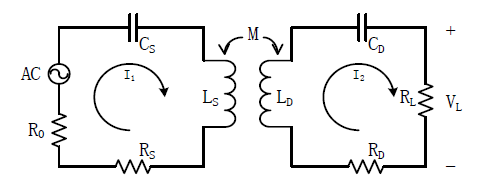Figure 1. Magnetic coupling resonant radio transmission system simplified model

$M={\mu }_{0}{n}_{1}{n}_{2}\cdot \sqrt{{r}_{1}\cdot {r}_{2}}\cdot b\cdot {\int }_{0}^{\frac{\text{π}}{2}}\frac{2{\mathrm{sin}}^{2}\theta -1}{\sqrt{1-{b}^{2}{\mathrm{sin}}^{2}\theta }}\text{d}\theta$ (3)

$M={\mu }_{0}{n}^{2}r\cdot \sqrt{\frac{4{r}^{2}}{4{r}^{2}+{D}^{2}}}\cdot {\int }_{0}^{\frac{\text{π}}{2}}\frac{2{\mathrm{sin}}^{2}\theta -1}{\sqrt{1-\frac{4{r}^{2}}{4{r}^{2}+{D}^{2}}{\mathrm{sin}}^{2}\theta }}\text{d}\theta$ (4)

$\frac{\omega M}{R}=1$ (5)

2.2.2. 系统效率特性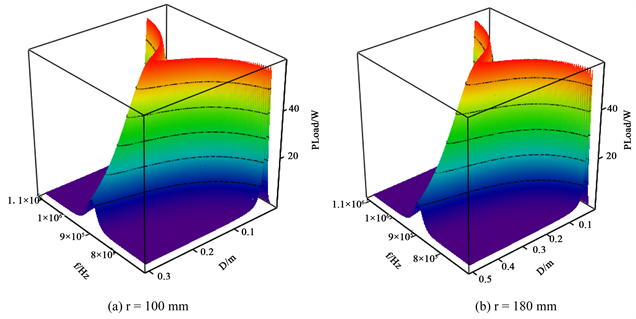Figure 2. Relational surface diagram of PLoad, ω, D

$\begin{array}{c}\eta =\frac{{P}_{L}}{{P}_{Total}}=\frac{{|{\stackrel{˙}{I}}_{2}|}^{2}{R}_{L}}{{|{\stackrel{˙}{I}}_{1}|}^{2}\left({R}_{0}+{R}_{S}\right)+{|{\stackrel{˙}{I}}_{2}|}^{2}\left({R}_{D}+{R}_{L}\right)}\\ =\frac{{\left(\omega M\right)}^{2}{R}_{L}}{\left[{\left({R}_{D}+{R}_{L}\right)}^{2}+{X}_{D}^{2}\right]\left({R}_{0}+{R}_{S}\right)+{\left(\omega M\right)}^{2}\left({R}_{D}+{R}_{L}\right)}\\ =\frac{{\left(\omega M\right)}^{2}{R}_{L}}{\left({R}_{2}^{2}+{X}^{2}\right){R}_{1}+{\left(\omega M\right)}^{2}{R}_{2}}\end{array}$ (6)

3. 线圈半径对磁耦合谐振式无线电能传输特性的影响

3.1. 线圈半径r对传输系统两线圈间互感M的影响

1) 线圈半径r对两线圈间的互感影响很大。在距离不变的情况下，线圈间的互感随线圈半径的增大而增大；

2) 随着距离的增大，线圈间的互感均单调减小。从而得到线圈半径r和传输距离D是影响系统互感的主要因素，这亦可从式(4)中看出。Figure 3. Relational surface diagram of PLoad, ω, D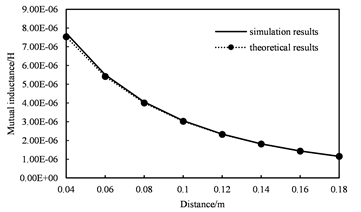(a) r = 100 mm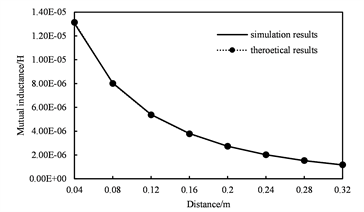(b) r = 180 mm

Figure 4. The relationship of mutual inductance and distance between two coils

3.2. 线圈半径r对系统输出功率的频率特性的影响

3.3. 线圈半径r对系统效率的距离特性的影响

1) 理论计算结果与有限元瞬态仿真结果具有完全一致性，从而验证了理论分析的正确性；

2) 随着传输距离的最大，系统效率均单z减小，且在同等传输距离的情况下，线圈半径较大的传输系统具有较大的效率；

3) 在同等传输效率情况下，线圈半径较大的传输具有更远传输距离的能力。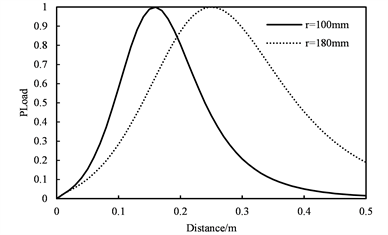Figure 5. The normalized output power varies with the distance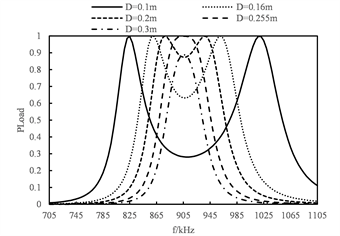(a) r = 100 mm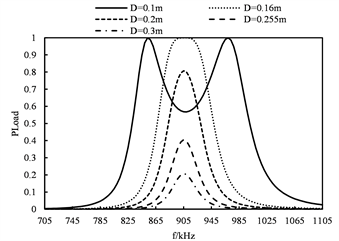(b) r = 180 mm

Figure 6. The normalized output power varies with the distance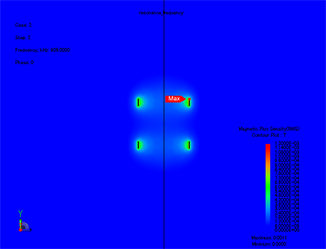(a) 线圈半径100 mm，距离为160 mm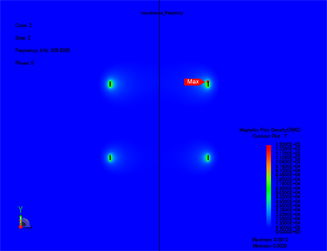(b) 线圈半径180 mm，距离为255 mm

Figure 7. Induced field distribution

4. 实验分析

5. 结论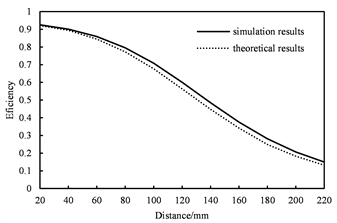(a) r = 100 mm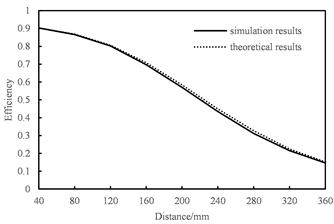(b) r = 180 mm

Figure 8. The system efficiency varies with the transmission distance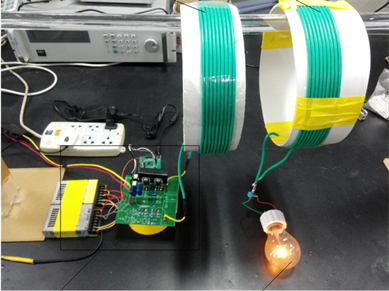Figure 9. The experimental device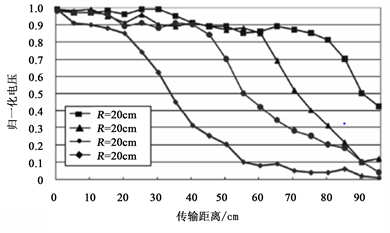Figure 10. The experimental waveforms

45 cm、60 cm、75 cm的距离处开始显著下降；在20 cm、45 cm、60 cm、75 cm的距离处负载上接收到的电压分别为最大值的 85%、85%、90%、85%。图中存在一定的误差主要是线圈手工绕制和忽略辐射损耗造成的，因此可以证明有效传输距离与线圈半径成正比。

  王振亚. 谐振式无线电能传输系统的优化设计[D]: [硕士学位论文]. 广州: 华南理工大学, 2015.  刘瑞. 小功率磁耦合谐振式无线电能传输特性及其实验研究[D]: [硕士学位论文]. 合肥: 合肥工业大学, 2015.  张鹏. 电磁耦合共振式无线电能传输系统的仿真与实验[D]: [硕士学位论文]. 吉林: 东北电力大学, 2015.  赵争鸣, 张艺明, 陈凯楠. 磁耦合谐振式无线电能传输技术新进展[J]. 中国电机工程学报, 2013, 33(3): 1-13.  杨庆新, 陈海燕, 徐桂芝, 等. 无接触电能传输技术的研究进展[J]. 电工技术学报, 2010, 25(7): 6-13.  Kaneko, Y. and Abe, S. (2013) Technology Trends of Wireless Power Transfer Systems for Electric Vehicle and Plug-In Hybrid Electric Vehicle. IEEE, 1009-1014. https://doi.org/10.1109/PEDS.2013.6527167  杨庆新, 章鹏程, 祝丽花, 等. 无线电能传输技术的关键基础与技术瓶颈问题[J]. 电工技术学报, 2015, 30(5): 1-8.  李炜昕, 王炅, 张合, 等. 基于磁耦合谐振的无线能量传输系统[J]. 系统工程与电子技术, 2014, 36(4): 637-642.  唐治德, 徐阳阳, 赵茂, 等. 耦合谐振式无线电能传输的传输效率最佳频率[J]. 电机与控制学报, 2015, 19(3): 8-13.